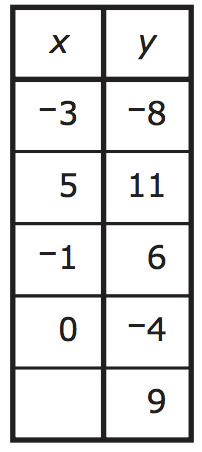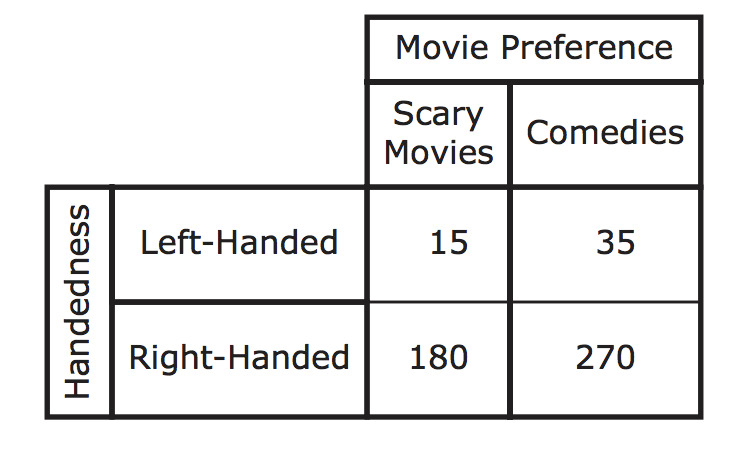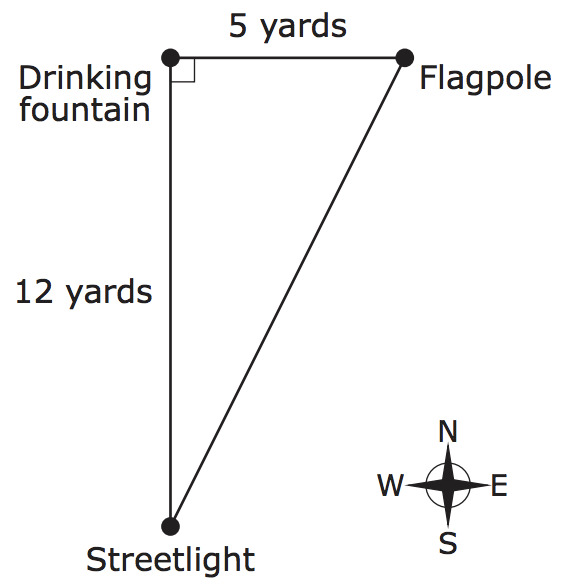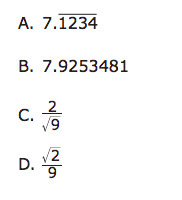PSA8QB5 - Grade 8 Mathematics Practice Test 2017

Rae made a table of x and y values. The relationship between the x and y values in Rae’s table is not a function. One of the values of x is missing from Rae’s table, as shown.What is one value of x that Rae could use to complete her table to show that the relationship between x and y is not a function?

Type your answer below as a number (example: 5, 3.1, 4 1/2, or 3/2):
Part A)

Jason is comparing the sizes of Earth, Saturn, and a lacrosse ball. The radius of Earth is approximately 6,378,100 meters.

Part A

What is the radius of Earth, in meters, written as a single-digit number multiplied by a power of 10? Enter your answer in the space provided. Enter only your answer.

Part B)

Part B

The radius of Saturn is approximately 6 × 107 meters.

Part C)

Part C

The radius of a lacrosse ball is approximately 0.032 meter.

Estimate the radius of a lacrosse ball, in meters, by expressing the radius as a single-digit number multiplied by a power of 10.

Part D)

Part D

Use your answers from Parts A and C to estimate how many times greater the radius of Earth is than the radius of a lacrosse ball. Show or explain how you got your answer.

A researcher surveyed 500 people and asked these two questions:

• Are you left-handed or right-handed?

• Do you prefer scary movies or comedies?

This table shows the results of the survey.Based on the results of the survey, what is the relative frequency of left-handed people who prefer comedies to all people who prefer comedies?

Select one:

In a park, the locations of a drinking fountain, a streetlight, and a flagpole form a right triangle. The drinking fountain is 12 yards north of the streetlight, and the flagpole is 5 yards east of the drinking fountain, as shown in this diagram.What is the shortest distance from the streetlight to the flagpole?

Select one:

Which of the following is an irrational number?Select one: